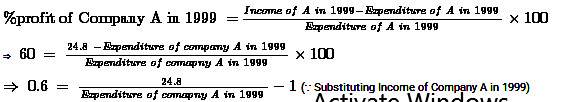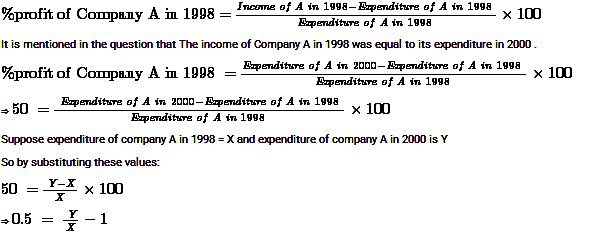# Data Interpretation Practice Questions – Set 87

Data Interpretation Practice Questions – Set 87
Directions (1 – 5): Below is given a pair of pie chart and bar graph representing number of students in different classes in a school in 2015 and growth in 2016. Based on this information, answer the questions that follow.
1. The students in 2016 in different classes are represented in a pie chart. What will be the difference between angles subtended by sectors representing 7thand 9th classes?(in°)
(a)  30
(b)  36.24
(c)  39.93
(d)  45
(e)  48.2
2. If the increment in all the classes has only been in the form of girl students, then what will be the ratio of total number of boys and girls in 2016?
(a)  43 : 41
(b)  23 : 27
(c)  8 : 9
(d)  2 : 3
(e)  Cannot be determined
3. Instead of 20%, what should be the minimum growth in number of students for class 7th, so that it does not have minimum number of students in 2016?
(a)  41.12
(b)  43.89
(c)  46.67
(d)  48
(e)  49.52
4. What will be the average number of students in classes 8th and 9th taken together in 2015 and 2016?
(a)  164
(b)  171
(c)  177
(d)  183
(e)  189
5. What should be growth in number of students for class 8th in 2017 so that their number becomes same as number of students in class 9th in 2016?
(a)  10%
(b)  12%
(c)  15%
(d)  8%
(e)  7.5%
Directions (6 – 10): Study the table carefully and answer the following question.
The given table represents 10th and 12th class students reading different subject in a school.
6. What reading the difference between the number of 10th class students reading English subject and the number of 12th class students reading Math?
(a)  532
(b)  680
(c)  482
(d)  702
(e)  None of these
7. Which subject has the maximum number of 10th class students reading?
(a)  Hindi
(b)  Physics
(c)  Chemistry
(d)  Computer
(e)  Economics
8. The 10th class students reading Hindi subject are how much percent more than 12th class students reading Hindi subject?
(a)  35%
(b)  48%
(c)  75%
(d)  55%
(e)  None of these
9. What is the approximate average number of 12th class students reading subjects in English language?
(a)  572
(b)  463
(c)  372
(d)  562
(e)  509
10. What is the difference between the number of 12th students reading computer and the number of 10th students reading economics?
(a)  432
(b)  558
(c)  468
(d)  238
(e)  None of these
Directions (11 – 15): Study the following graphs carefully and answer the questions that follow.
Percentage profit earned by two companies over the given years
% Profit = (Income – Expenditure)/Expenditure × 100
11. If the total income of Company A in all the years together was equal to the total expenditure of Company B in all the years together, which was Rs. 265 lakhs, what was the total percentage profit earned by Company A for all the years together?
(a)  45
(b)  37
(c)  52
(d)  Cannot be determined
(e)  None of these
12. If the income of Company A in 1999 was equal to the expenditure of Company B in 2001, then what was the ratio of expenditure of Company A in 1999 to the income of Company B in 2001?
(a)  25: 56
(b)  66: 25
(c)  10: 13
(d)  13: 10
(e)  None of these
13. If the total expenditure of the two Companies in 2000 was Rs. 18 lakhs and expenditure of Companies A & B in that year were in the ratio of 4: 5 respectively, then what was the income of Company B in that year (in lakh)?
(a)  8
(b)  10
(c)  10.4
(d)  Cannot be determined
(e)  None of these
14. If the income of Company B in 1999 was Rs. 18.6 lakhs and ratio of incomes of Companies A % B in 1999 was 4: 3, What was the expenditure of company A in 1999 (in Rs. lakh)?
(a)  12.5
(b)  14.5
(c)  15.5
(d)  16.5
(e)  None of these
15. If the income of Company A in 1998 was equal to its expenditure in 2000, what was the ratio between Company’s expenditure in the years 1998 and 2000 respectively?
(a)  3: 2
(b)  2: 3
(c)  1: 2
(d)  Cannot be determined
(e)  None of these

Solutions:
(1 – 5): Explanation:
Number of students in class 6th in 2016 = 60 × (1 + 10/100) = 66
Number of students in class 7th in 2016 = 45 × (1 + 20/100) = 54
Number of students in class 8th in 2016 = 75 × (1 + 20/100) = 90
Number of students in class 9th in 2016 = 90 × (1 + 10/100) = 99
Number of students in class 10th in 2016 = 120 × (1 + 15/100) = 138
1. B) difference between angles subtended by sectors representing 7th and 9thclasses = [(99 - 54)/(66 + 54 + 90 + 99 + 138)] × 360° = 36.24°

2. E) We do not have the original distribution of boys and girls in 2015. So, actual ratio cannot be determined neither for 2015 and nor for 2016 after increment.
Ratio cannot be uniquely determined.

3. C) To not have the minimum value, number of students for class 7th should be more than 66.
Minimum growth = [(66 - 45)/45] × 100 = 46.67%

4. C) average number of students in classes 8th and 9th taken together in 2015 and 2016 ((75 + 90) + (90 + 99))/2 = 177

5. A) Suppose growth is T% for class 8th students in 2017.
90 × (1 + T/100) = 99
T = (99/90 - 1) × 100 = 10
Growth should be 10%.

6. B) It is given that number of 10th class students reading English subject
= 9/13 × 1352 = 936
Number of 12th class students reading Math’s = 1/4 × 1024 = 256
Required difference = 936 256 = 680

7. B) Given that, number of 10th class students reading Physics
= 4/7 × 2401 = 1372
Number of 10th class students reading Chemistry = 2/3 × 729 = 486
Number of 10th class students reading Hindi = 7/11 × 1331 = 847
Number of 10th class students reading Computer = 7/10 × 1500 = 1050
Number of 10th class students reading language = 4/13 × 2197 = 676
Number of 10th class students reading Math = 3/4 × 1024 = 768
Number of 10th class students reading Economics = 7/12 × 1728 = 1008
Number of 10th class students reading English = 9/13 × 1352 = 936
Since, the 10th class students are reading in maximum for Physics. Therefore this subject has the maximum percentage of 10th class students.

8. C) It is given that number of 10th class students reading Hindi = 7/11 × 1331 = 847
Number of 12th class students reading Hindi = 4/11 × 1331 = 484
Therefore required percentage = (847 – 484)/484 × 100 = 363/484 × 100 = 75%

9. A) Required Average = (1029 + 243 + 450 + 720 + 416)/5 = 2858/5 = 571.6 ≈ 572

10. B) According to question, number of 12th class students reading computer
= 3/10 × 1500 = 450
Number of 10th class students reading economics = 7/12 × 1728 = 1008
Required difference = 1008 450 = 558

11. D) The answer can’t be determined. Because we need more data to solve this type of question.
We don’t have the Expenditure of all over the year for company A that is essential to solve this question.

12. A) Suppose Income of Company A in 1999 = X
Therefore from the question, the expenditure of Company B in 2001 = X
Also Expenditure of company A in 1999 = Y
And Income of company B in 2001 = Z0.4 = Z/X - 1
1.4 = Z/X
0.714 = X/Z
Ratio between the Expenditure of company A in 1999 to income of company B in 2001 is
= Y / Z
= (Y/X) × (X/Z)
= 0.625 × 0.714           ( Substituting Values of Y/Z and X/Z))
= 0.446
= 25 / 56

13. E) The total expenditure of the two Companies in 2000 was Rs. 18 lakhs
Expenditure of Companies A & B in that year were in the ratio of 4: 5
If ratio factor is X lakhs then
Expenditure of Company A = 4X lakhs
Expenditure of Company B = 5X lakhs
Total Expenditure = 18 lakhs
4X + 5X = 18
9X =18
X = 2
Expenditure of Company A = 4 × X = 4 × 2 = 8 lakhs
Expenditure of Company B = 5 × X = 5 × 2 = 10 lakhs
Profit percentage of company B in 2000 = 60 %14. C) Income of Company B in 1999 was Rs 18.6 lakhs
Ratio of incomes of Companies A and B in 1999 was 4: 3
Income of company B in 1999 = 18.6 lakhs
Income of company A in 1999/Income of company B in 1999 = 4/3
Income of company A in 1999 = 4 × 18.6 /3
Income of company A in 1999 = 24.8 lakhs
Profit percentage of company A in 1999 = 60 %1.6 = 24.8 / Expenditure of company A in 1999
Expenditure of company A in 1999 = 24.8 / 1.6
Expenditure of company A in 1999 = 15.5 lakhs

15. B) Percentage of profit of company A in 1998 is 50%
Percentage profit earned by two companies over the given years
By the formula we get,1.5 = Y/X
X/Y = 1/1.5
X/Y = 0.666
X/Y = 2/3